# Production is given by p(x, y) = 10x(y^2) + x^2 inputs are restricted by x=(z^3) and...

Production is given by

p(x, y) = 10x(y^2) + x^2

inputs are restricted by

x=(z^3) and y=(2z^2)

using the chain rule what is the production rate of change when z=1?

What is the isoquant and marginal rate of technical substitution when x=2 and y=3.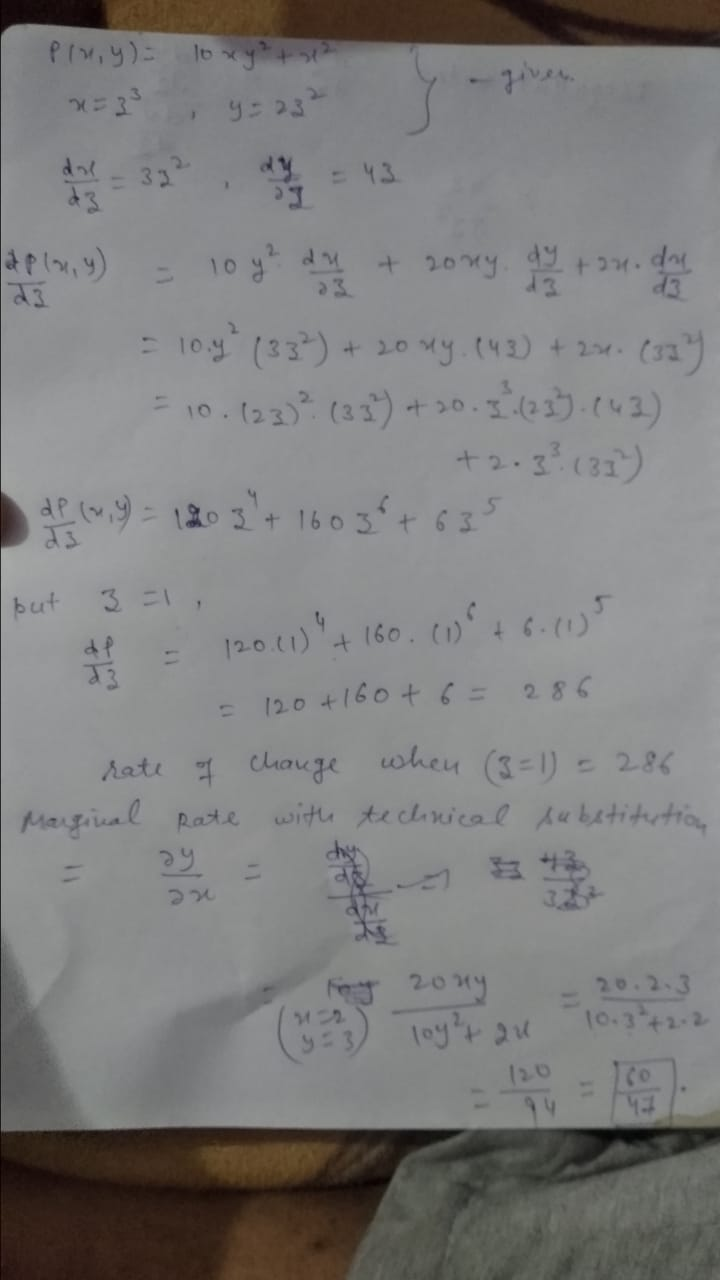##### Add Answer of: Production is given by p(x, y) = 10x(y^2) + x^2 inputs are restricted by x=(z^3) and...
Similar Homework Help Questions
• ### 3. Consider the following production function with two inputs X1 and x2. y = alnx +...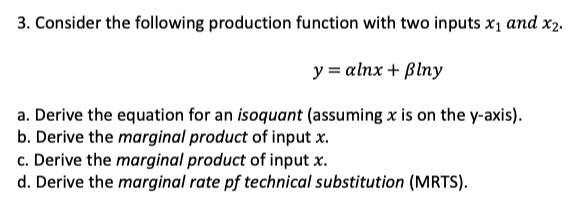3. Consider the following production function with two inputs X1 and x2. y = alnx + Blny a. Derive the equation for an isoquant (assuming x is on the y-axis). b. Derive the marginal product of input x. c. Derive the marginal product of input x. d. Derive the marginal rate pf technical substitution (MRTS).

• ### 2. Consider the following production function with two inputs X1 and X2. y = x1/2x2/4 a....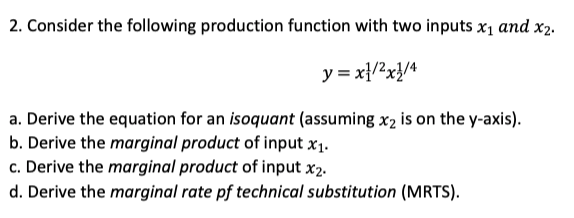2. Consider the following production function with two inputs X1 and X2. y = x1/2x2/4 a. Derive the equation for an isoquant (assuming X2 is on the y-axis). b. Derive the marginal product of input x1. c. Derive the marginal product of input x2. d. Derive the marginal rate pf technical substitution (MRTS).

• ### TUTORIAL2 Chapter 7 Part 1 Key Concepts and Equations: Production Isoquant: shows all combinations of input...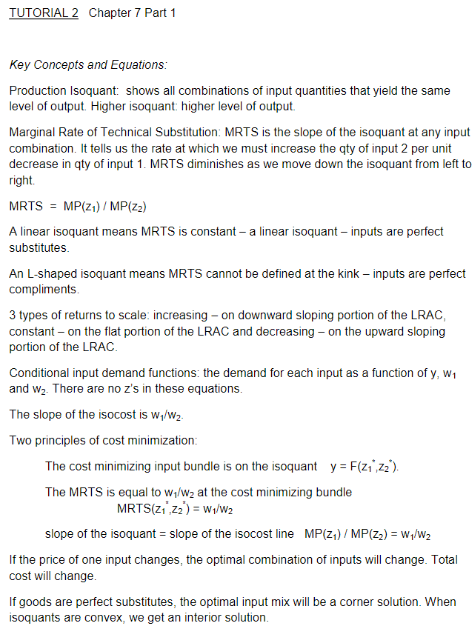TUTORIAL2 Chapter 7 Part 1 Key Concepts and Equations: Production Isoquant: shows all combinations of input quantities that yield the same level of output. Higher isoquant: higher level of output Marginal Rate of Technical Substitution: MRTS is the slope of the isoquant at any input combination. It tells us the rate at which we must increase the qty of input 2 per unit decrease in qty of input 1. MRTS diminishes as we move down the isoquant from left to...

• ### 3. Let q = Lākā denote the production function for a firm making long-run decisions, that...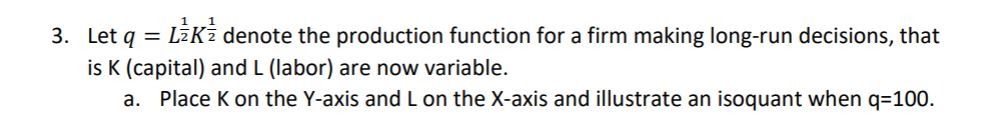3. Let q = Lākā denote the production function for a firm making long-run decisions, that is K (capital) and L (labor) are now variable. a. Place k on the Y-axis and L on the X-axis and illustrate an isoquant when q=100. b. Derive an expression for the MRTS (the marginal rate of technical substitution) for any level of q.

• ### Consider the function f(x,y,z) = 2 4 4 3 63 - 26 x y z - 8 y z + 76 x + 18 y - 31 y z . Find (a)...

Consider the function f(x,y,z) =63 - 26 x y z - 8 y^2z + 76 x^4+ 18 y^4- 31 yz^3.Find (a) the greatest rate of change of f atP=(19, 13, 4), and (b) the UNIT vector that points inthe direction of greatest rate of change of f atP

• ### Given production function: y=f(x1,x2)=(α⋅x(σ−1)/σ1+(1−α)⋅x(σ−1)/σ2)σ/(σ−1) consider,   α = 0.2 and σ = 0.7. The first factor is...

Given production function: y=f(x1,x2)=(α⋅x(σ−1)/σ1+(1−α)⋅x(σ−1)/σ2)σ/(σ−1) consider,   α = 0.2 and σ = 0.7. The first factor is currently used in the amount x1 = 9, and the second factor is used in the amount x2 = 3.   a) When (x1,x2) = (9,3), how much output is being produced? Output:     b) When (x1,x2) = (9,3), what is the marginal product of factor 1? Marginal product:    c) When (x1,x2) = (9,3), what is the average product of factor 1? Average product:     d) When...

• ### a - e (a) X + y +z = 11 X – Y – 2= -3...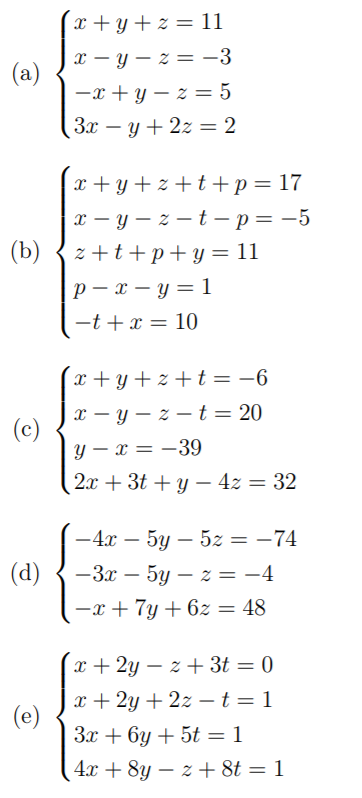a - e (a) X + y +z = 11 X – Y – 2= -3 -2 + y - 2 = 5 (3x – y + 2z = 2 (b) x+y+z+t+p=17 X - Y - 2-t-p= -5 z +t+ p + y = 11 p - x - y = 1 -t + x = 10 (c) x +y + 2+t= -6 X - Y - 2 -t = 20 y - X=-39 2x + 3t + y -...

• ### Find the directional derivative of the function f(x,y,z) = -67 y^2 z + 8 z^3 - 48 x^2 y z - 25 x...

Find the directional derivative of the function f(x,y,z) = -67 y^2z + 8 z^2- 48 x^2y z - 25 x y^2z+ 93 x^4yat the pointP= (6, -14, -7) inthe direction fromPto the pointP=(14, 3, 16)

• ### TUTORIAL2 Chapter 7 Part 1 Key Concepts and Equations: Production Isoquant: shows all combinations of input...TUTORIAL2 Chapter 7 Part 1 Key Concepts and Equations: Production Isoquant: shows all combinations of input quantities that yield the same level of output. Higher isoquant: higher level of output Marginal Rate of Technical Substitution: MRTS is the slope of the isoquant at any input combination. It tells us the rate at which we must increase the qty of input 2 per unit decrease in qty of input 1. MRTS diminishes as we move down the isoquant from left to...

• ### 4. Consider the production functions given below: a. Suppose that the production function faced by a...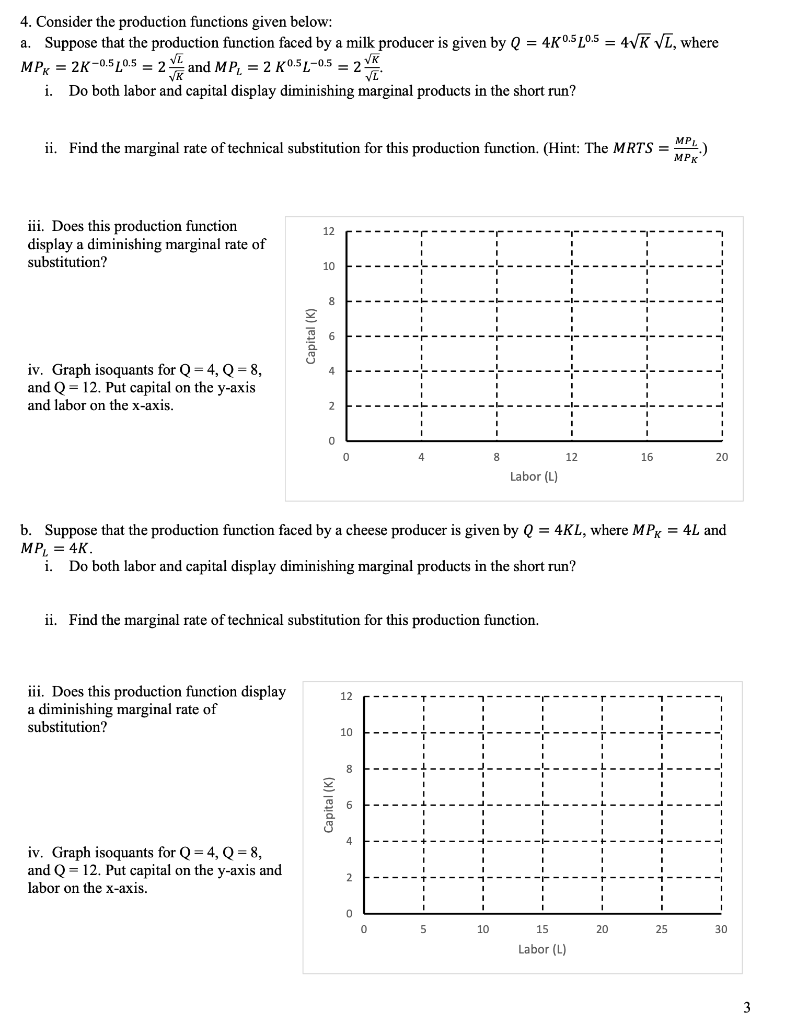4. Consider the production functions given below: a. Suppose that the production function faced by a milk producer is given by Q = 40.5 20.5 = 4VK VL, where MPx = 2K-0.5 20.5 = 2 and MP, = 2 K0.5L-05 = 2 * i. Do both labor and capital display diminishing marginal products in the short run? ii. Find the marginal rate of technical substitution for this production function. (Hint: The MRTS = 1) iii. Does this production function display...

Free Homework App